#2018 iT 邦幫忙鐵人賽DAY 30
0
Software Development

# 協定類型的集合

``````protocol AfuncProtocol {
func msg()
}

class Printer:AfuncProtocol {
func msg() {
print("鐵人30天完成")
}
}

let printer1 = Printer()
let printer2 = Printer()
let printer3 = Printer()

let printers:[AfuncProtocol] = [printer1,printer2,printer3]

for printer in printers {
printer.msg()
}
``````# 協定的繼承

``````protocol InheritingProtocol: SomeProtocol, AnotherProtocol {
// 協定內容
}
``````

# Class 專用的協定

``````protocol SomeClassOnlyProtocol: AnyObject, SomeInheritedProtocol {
// class 專用的協定內容
}
``````

# 協定組合

``````protocol Groups {
var groups:String {get}
}

protocol Names {
var names:String {get}
}

struct Person:Groups, Names {
var groups: String
var names: String
}

func celebrate(to celebrate: Groups & Names) {
print("恭喜「 \(celebrate.groups) 」的成員 \(celebrate.names)，完成「 30天鐵人競賽」 ！")
}

let ironMan = Person(groups: "好想工作室", names: "Jeremy")
celebrate(to: ironMan)
``````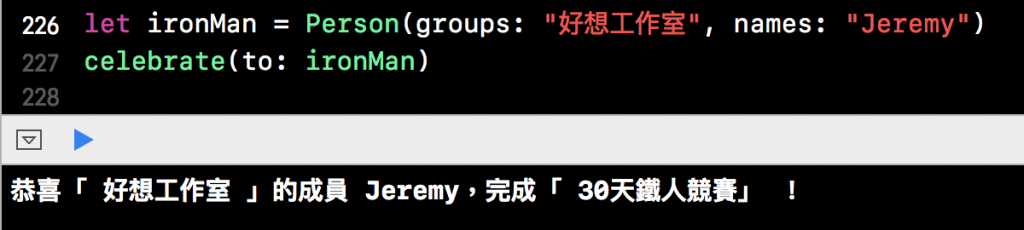# 協定遵循的檢查

• 如果實例遵循協議，那麼 is 運算符就會返回 true，反之則返回 false
• as ? 向下轉換運算符返回協議的可選項，如果遵循協議就會返回協定類型的可選值，反之則返回nil。
• as ! 向下轉換運算符強制轉換協議類型並且在失敗是觸發運行錯誤。

``````protocol Area {
var area:Int {get}
}
``````

``````class Square:Area {
var area: Int
init(width:Int) {
self.area = width * width
}
}
class Triangle:Area {
var area: Int
init(width:Int,height:Int) {
self.area = width * height / 2
}
}
``````

``````class Cube {
var volume:Int
init(width:Int,length:Int,height:Int) {
volume = width * length * height
}
}
``````

``````let square = Square(width: 10)
let triangle = Triangle(width: 10, height: 10)
let cube = Cube(width: 20, length:10 , height: 10)

let areas:[AnyObject] = [square,triangle,cube]
``````

``````let areas:[AnyObject] = [Square(width:10),Triangle(width:10,height:10),Cube(width:10,length:10,height:5)]
``````

``````for area in areas{
if let haveArea = area as? Area {
print("\(haveArea.name)面積為：\(haveArea.area)")
} else {
print("不遵循 Area 協定，無法計算面積")
}
}
``````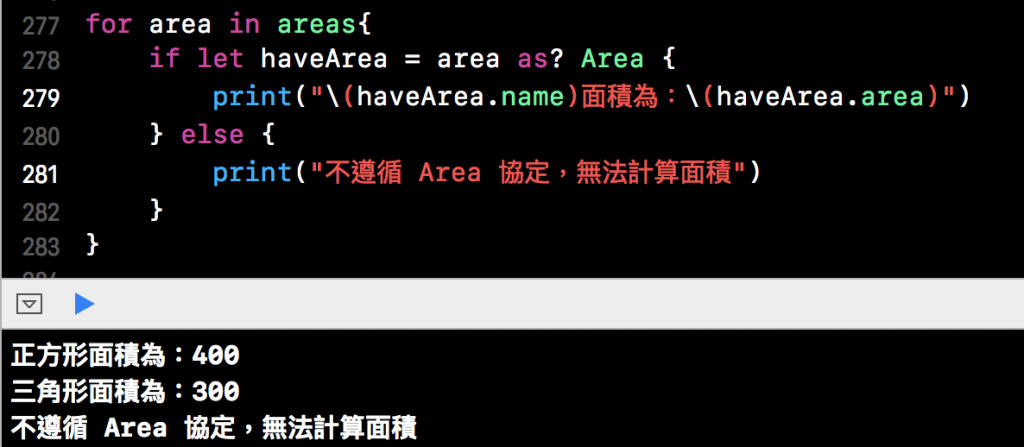# 可選協定要求

``````import Foundation
//這邊發現必須 import Foundation 才能使用可選協定，好像是因為可選協定與 Objective-C 程式語言有關係，而 Objective-C 大量使用到 Foundation 的功能 所以需要 import，調查中...。

@objc protocol OptionalProtocol {
@objc optional func add() -> Int
@objc optional func sub() -> Int
}
``````

``````var a = 5
var b = 10

class Math:OptionalProtocol {

@objc func add() -> Int {
return a + b
}

// 注意，這邊我們沒有編寫 sub()方法
}
``````

``````var math:OptionalProtocol = Math()

if let result = math.sub?() {
print(result)
}

if let result = math.add?(){
print(result)
}
``````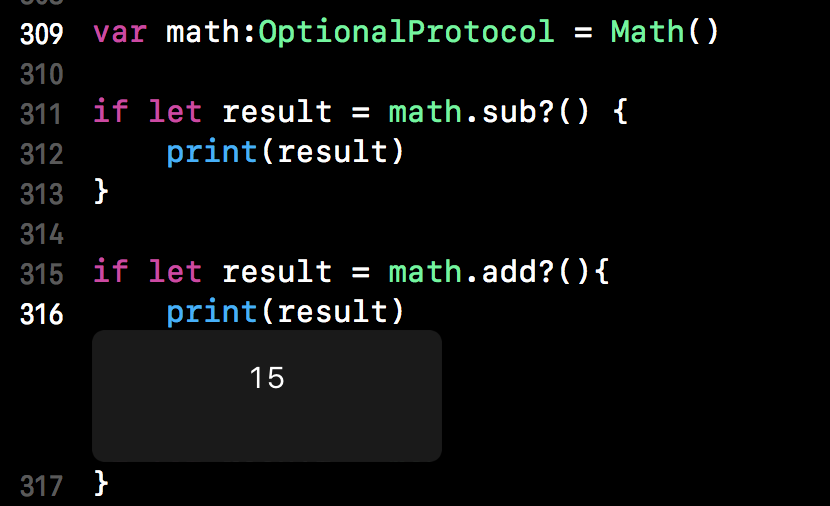# 協定擴展

``````var result = 0
var a = 5
var b = 10

protocol Math {
func math()
}

class Add:Math {
func math() {
result = a + b
}
}
``````

``````extension Math {
func printer() {
print("a + b 結果為：\(result)")
}
}
``````

``````let someAdd = Add()
someAdd.math()
someAdd.printer()
``````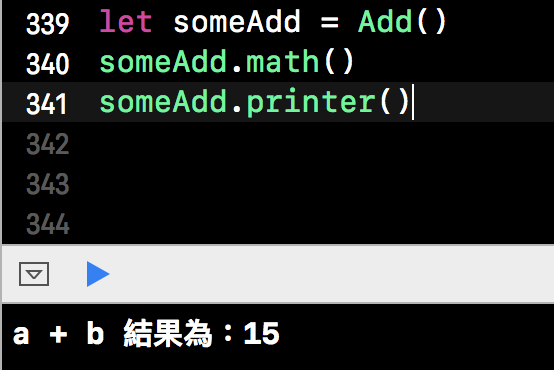## 提供默認實現

``````protocol DefaultProtocol {
var name:String {get}
}

extension DefaultProtocol {
var msg:String {
return "默認字串"
}
}
``````
``````class Msg:DefaultProtocol {
var name = "Jeremy"
}

let defaultTest = Msg()
defaultTest.name
defaultTest.msg
``````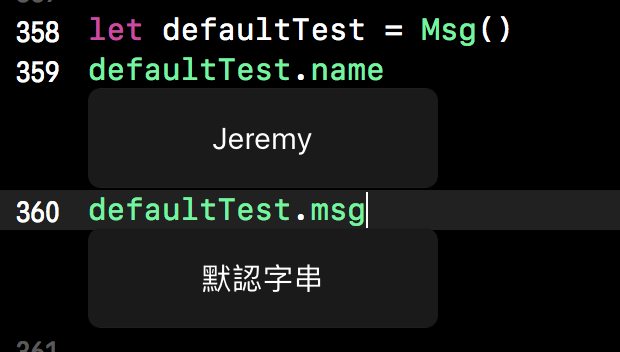Swift 菜鳥的30天30